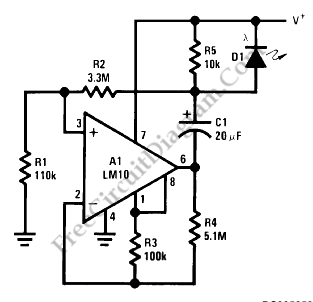# Low Power Under- and Over-Voltage MonitorThis voltage monitor has two  threshold, VTH for undervoltage and VTH’ for overvoltage. Using the component values shown in the schematic diagram below, this circuit give 6V for VTH and 15V for VTH1. Above 6V, the LED indicator of this voltage monitor  circuit will increase the flash rate until reach 15V.  This circuit will stop flashing at voltage below 6V and above 15 volts since there will be no current flowing through C1.  At threshold  boundary, the output of LM10 will saturate to negative below VTH and saturate to positive above VTH’.To customize circuit we can select the resistors values according to chosen VTH and VTH’ using the following formula:

VTH=[R4(R1+R2)Vref]/[R1(R3+R4)];

VTH’=[R4(R1+R2)Vref]/[R1(R3+R4)-R3(R1+R2)]

Since the current consumption is very small (around 500uA), this voltage monitor will be suitable for various application  demanding low cost solution,  such as battery monitoring, small testing equipments, or power line indication.  [Circuit’s schematic diagram source: National Semiconductor Application Note]